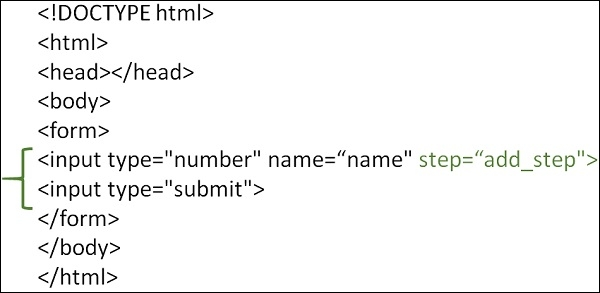# How to use different step attribute in one range input in HTML?

HTMLWeb DevelopmentFront End Technology

The HTML input type step attribute sets the legal number intervals. Steps are number steps like 0, 2, 4, 6, 8, 10, etc. The step attribute can be used together with the max and min attributes to create a range of legal values.

To use different step attribute in one range input in HTML, we will use JavaScript. First, let’s see how to use step attribute.You can try to run the following code to create a step of numbers with the input step attribute. Here, we are creating a step 2, so the valid input will be 2, 4, 6, 8, 10, etc. On entering a number except that and pressing submit button, the following message will be visible: “Please enter a valid value”. It will also let you know the two nearest values.

## Example

Live Demo

<!DOCTYPE html>
<html>
<title>HTML input step attribute</title>
<body>
<form action = "/cgi-bin/hello_get.cgi" method = "get">
<input type = "number" name = "points" step = "2"><br>
<input type = "submit" value = "Submit">
</form>
</body>
</html>

We will use jQuery to provide different step attribute in one range input in HTML. Here, we have added two steps i.e. 10 and 2. The range here is from 100 to 450, which is set under min and max attribute.

## Example

Live Demo

<html>
</script>
<script type="text/javascript">
$(function() {$('#points').on('input change', function() {
var element = $('#points'), value = element.val(), step; // Set rules here if (value < 205) { step = 10; }else { step = 2; } element.attr('step', step);$('#value').text(value);
\$('#step').text(step);
});
});
</script>

<body>
<div>
Value: <span id="value"></span>
</div>
<div>
Current step: <span id="step"></span>
</div>
<div>
<input id="points" type="range" value="100" min="100" max="450" />
</div>
</body>
</html>